Courses

# Fresnel's Law - EM Waves, Electromagnetic Theory, CSIR-NET Physical Sciences Physics Notes | EduRev

## Physics for IIT JAM, UGC - NET, CSIR NET

Created by: Akhilesh Thakur

## Physics : Fresnel's Law - EM Waves, Electromagnetic Theory, CSIR-NET Physical Sciences Physics Notes | EduRev

The document Fresnel's Law - EM Waves, Electromagnetic Theory, CSIR-NET Physical Sciences Physics Notes | EduRev is a part of the Physics Course Physics for IIT JAM, UGC - NET, CSIR NET.
All you need of Physics at this link: Physics

Fresnel's equations describe the reflection and transmission of electromagnetic waves at an interface. That is, they give the reflection and transmission coefficients for waves parallel and perpendicular to the plane of incidence. For a dielectric medium where Snell's Law can be used to relate the incident and transmitted angles, Fresnel's Equations can be stated in terms of the angles of incidence and transmission.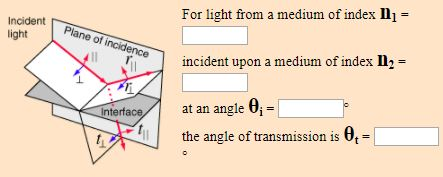Fresnel's equations give the reflection coefficients: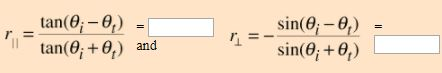The transmission coefficients are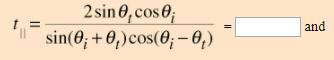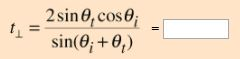Note that these coefficients are fractional amplitudes, and must be squared to get fractional intensities for reflection and transmission. The signs of the coefficients depend on the original choices of field directions.

You can choose values of parameters which will give transmission coefficients greater than 1, and that would appear to violate conservation of energy. (For example, try light incident from a medium of n1=1.5 upon a medium of n2=1.0 with an angle of incidence of 30°.) But the square of the transmission coefficient gives the transmitted energy flux per unit area(intensity), and the area of the transmitted beam is smaller in the refracted beam than in the incident beam if the index of refraction is less than that of the incident medium. When you take the intensity times the area for both the reflected and refracted beams, the total energy flux must equal that in the incident beam. For further details, see Jenkins and White.

Checking out conservation of energy in this situation leads to the relationship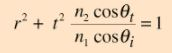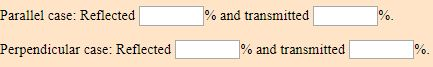Reflection and Transmission

Typical reflection and transmission curves for external reflection. These curves are the graphical representation of the Fresnel equations. Note that the reflected amplitude for the light polarized parallel to the incident plane is zero for a specific angle called the Brewster angle. The reflected light is then linearly polarized in a plane perpendicular to the incident plane. This polarization by reflection is exploited in numerous optical devices.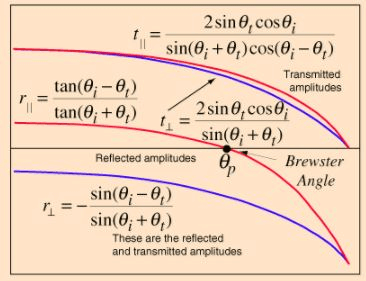Internal Reflection Curves

The illustration shows typical reflection curves for internal reflection. Internal reflection implies that the reflection is from an interface to a medium of lesser index of refraction, as from water to air. These curves are the graphical representation of the Fresnel equations. Note that the reflected amplitude for the light polarized parallel to the incident plane is zero for a specific angle called the Brewster angle. The reflected light is then linearly polarized in a plane perpendicular to the incident plane. This polarization by reflection is exploited in numerous optical devices. Another characteristic of internal reflection is that there is always an angle of incidence qc above which all light is reflected back into the medium. This phenomenon of total internal reflection has many practical applications in optics.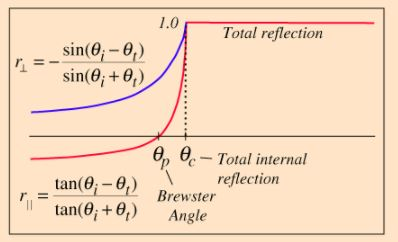159 docs

,

,

,

,

,

,

,

,

,

,

,

,

,

,

,

,

,

,

,

,

,

,

,

,

,

,

,

;